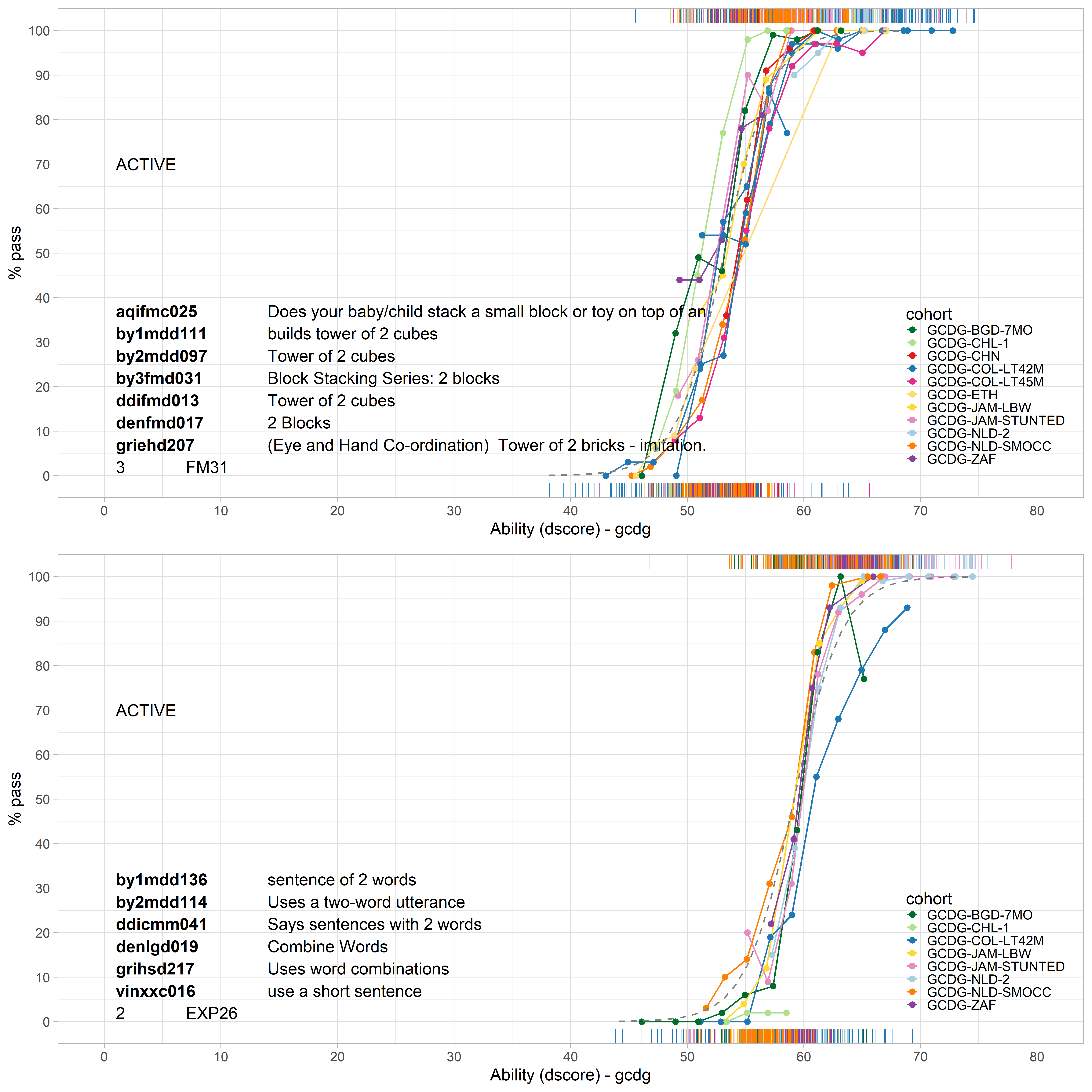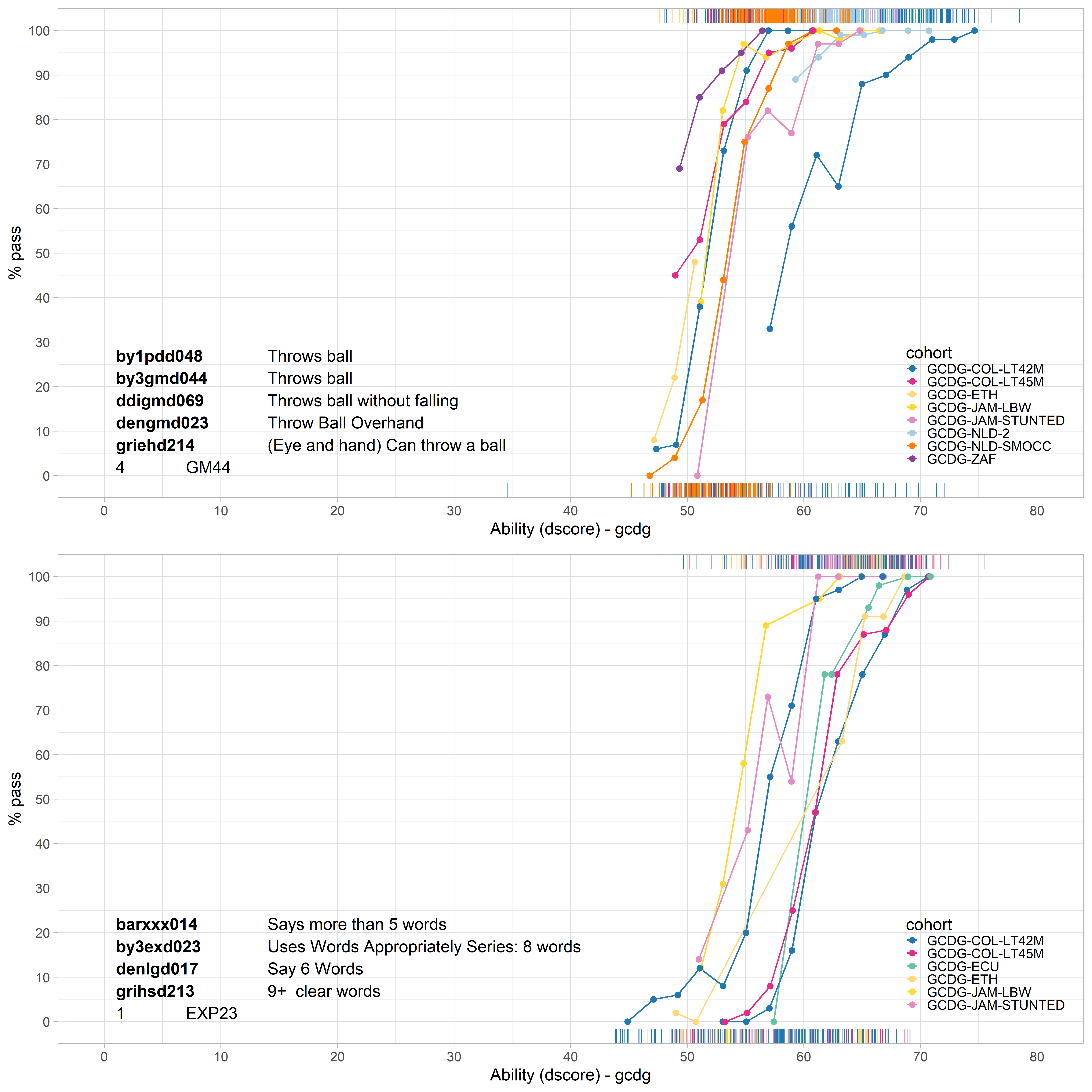## 4.7 Differential item functioning

Items within an active equate group should work in the same way across the different cohorts, i.e., they have no differential item functioning (DIF). The assumption of no DIF is critical for active equate groups. If violated, restricting the difficulty parameters as equal across cohorts may introduce unwanted bias in comparisons between cohorts. This section illustrates the role of DIF in equate groups.

### 4.7.1 Good equate groups without DIF

Chapter I discusses the role of DIF in the evaluation of the fit of items to the Rasch model. This section illustrates similar issues in the context of equate groups.Figure 4.6: Two equate groups that present no differential item functioning between cohorts.

Figure 4.6 shows the empirical curves of two equate groups, FM31 (two cubes) and EXP26 (two-word sentence). All curves are close to each other, so there is no differential item functioning here.

### 4.7.2 Poor equate groups with DIF for studyFigure 4.7: Two equate groups that present differential item functioning between cohorts.

Figure 4.7 plots the empirical curves for equate groups GM44 (throws ball) and EXP23 (5 or more words). The substantial variation between these curves is a sign of differential item functioning. For example, Throws ball is easier for children in the South-Africa cohort (purple curve; GCDG-ZAF) and more difficult for children in Colombia (blue curve; GCDG-COL-LT42M). In other words, the probability of passing the item given the D-score (i.e. item difficulty) differs between the cohorts. Likewise, there is differential item functioning for Says more than 5 words. This milestone is easier for children in Jamaica (yellow and pink curves; GCDG-JAM-LBW and GCDG-JAM-STUNTED) than for children from Ecuador (green; GCDG-ECU).